The Mechanism of Reality

By Robert Dudley Berne

No reproductions are permitted without the written permission of Robert Dudley Berne

# Overview

## Preface

This paper uses a logical reasoning process to identify the underlying mechanism responsible for the laws of physics and describes the mechanism and the phenomena that manifest the laws.

The paper also presents an analysis of past physics theories about gravity, mass, and inertia. Many scientists since the 15th century have worked to develop perspectives on relativity. The relativity principle is important because it has repeatedly advanced scientific understanding of the physical world. This paper refers to the work of scientists who advanced physics theories including Copernicus, Hendrik Lorentz, Albert Einstein, Sir Isaac Newton, Wolfgang Pauli, Neils Bohr, Max Planck, and Hans Orsted. The paper examines briefly what is known about gravity, mass and inertia, and explains how an underlying mechanism presented as a new model creates observable effects and explains otherwise unexplained phenomena.

This document presents brief descriptions of potential future applications of this simple mechanism to enlighten the reader about the potential for applying the mechanism to contribute to science and engineering.

I have been looking at the issue of "the why and how" with regards to physics for my whole life. In particular, gravity. The space-time curvature model is incomplete as it never shows two bodies and their respective gravity wells and how those wells can come together. Simply adding a ball and saying that it rolls into the well is inconsistent with the model. What causes the ball to roll into the well? Ah, that would be gravity. So it is really only a poetic way of describing Newton's equation. I am not arguing the validity of the curvature math, only its applicability to a cause. It says how much, not why.

The model also violates the whole premise of relativity, that of a two or more body relationship.

I received a copy of Scientific American's February 2014 issue and the cover story, 'The Proton Problem', which described the difference in the proton's diameter when it is orbited by a muon. It is slightly but measurably smaller than when orbited by an electron.

The muon has an interesting mass loss of 99.5% when it decays into an electron; the resulting two neutrinos must have the energy difference. This got me thinking. And exploring. It appears that neutrinos are a result from multiple decay modes of many particles.

The role neutrinos play in physics has been clearly understated. If they are as prolific and as numerous as they are estimated to be, it is apparent that the neutrino is the elephant in the lab.

Neutrinos have been ignored far too long. Neil's DeGrasse Tyson on his televised series 'Cosmos' stated that the universe was born of a little golf ball marble of neutrinos.

It is like a great archeological architect, Dr. Hawking, found the tattered and worn blue prints that indicate brick construction; Dr. Tyson has found the kiln and Pauli the slurry. Dirac has found the bill of sale for the bricks. The demolition team at the LHC finds pieces of bricks all over the place after vaporizing the house---and yet---main stream physics refuses to accept the simple conclusion that the house is made of bricks.

For reasons I cannot even begin to understand, it hit me that if neutrinos permeate matter, they must refract and, if they do, when two massive bodies shadow each other from the normal flow, this refraction must push the bodies together. And that is gravity. Then it became apparent that if this were true, a body in motion would encounter the same refraction and more so as velocity increased, much like the Lorentz transformation with respect to apparent mass.

Then the bubble popped. Inertia. If this were true about refraction, then all matter would cease to move eventually. Game over.

Inertia ... a body in motion remains in motion. Then it came to me. If neutrinos make strings that are moving at the speed of light. Like an optical current. And what happens when a current is in a coil? It remains steady until something acts on it. If neutrino strings formed loops when they encountered matter, then they would form coils, like inductors, except for the difference that the 'wire' is moving at the speed of light. Although this is a difficult visualization, it works for the explanation of inertia, and also explains the basis of neutrino refraction.

So, we're back in the game. What happens when the coil that was encountering matter in motion from the side slips off the back? It uncoils making a wake or a drag. That is the inertial frame drag in the general theory of relativity. But it takes time to uncoil. And also space. On a small scale, like a hydrogen model, what little coiling an electron causes requires a smaller space when compared to the uncoiling space required when encountering a 200 times larger body like a muon. If the residual coiling were to encounter the central proton, it might compress it a bit and therefore make the proton's diameter smaller.

This explains the mechanism of the Proton Problem's Solution, as well as the rest of the physical phenomena that have defied explanation. While this work is light on the math, the next one will be focused on engineering solutions based on this simple theory of neutrinos and their strings. It will include a foundation that was started by Dirac almost 100 years ago.

But for now, I share with you the incredible journey into the mechanism of reality.

## Introduction

Reason is answering why, how, and possibly who from observations of where, what and when. Reason is valuable when there is little experience and questionable judgment. Reason also can build a framework of cause and effect and enable planning. It can be used to categorize concepts and facts; the resulting groupings can represent abstractions.

Using reason, it is possible to create a systematic model. Often, using reasoning to solve a problem without preconceptions about it is a better process than making assumptions about the solution based solely on experience. Reasoning helps those making inquiries to perceive the realm of possibilities. It also allows elimination of obvious but false solutions and impossible options.

The most successful method of solving a problem generally starts with a clear and valuable discussion that identifies the problem. Stating what something is not can be valuable, as well. This type of exercise defines boundaries. The process also defines what should be examined when the list of possibilities is completed.

Consistencies have never exposed a flaw in judgment. Instead, they shape a model. Inconsistencies are valuable because they can jar researchers' reasoning about situations and spark investigation. Inconsistencies can be used to reach two outcomes when studying a model hypothesis:

1. Enrich understanding of the problem, or

2. Remove the model hypothesis from the realm of possibilities.

It is the exception to a generalization that stimulates thinking, refines theories, and leads to discarding infeasible theories. Copernicus proposed what is considered the original theory of relativity when he identified the sun as the inertial center of the solar system, an observation considered revolutionary in the 15th century. He and his successors adopted inertia as the prime organizing principle for the understanding and description of nature based on exploring facts with reason. Note that Albert Einstein proposed the Special Theory of Relativity based on what he perceived as a departure from the expected results of the Michelson-Morley experiment regarding the speed of light conducted in 1887.Einstein said that eventually thought would take the place of lab work when solving problematical puzzles. With that statement, he made it clear that he valued reason over experience.

# Physics and Gravitational Force

## Reasoning and physics

One persistent physics puzzle is determining what causes gravity. There is a great deal of experience with this topic, judgments about this question, and reasoning contributed by scientists, researchers, and academics.

Observable relationships are part of experience. The work of Sir Isaac Newton is relevant to a discussion of gravity. He was the first to state mathematically the relationship between mass effort velocity and gravity. It is an exact observable relationship. The two givens in this case, velocity and effort, are well understood because they are defined by observations. However, though mass and gravity can be and have been measured, the measurement offers no explanation of what they are or what causes them.

This dilemma provides a path for this paper which examines the question using reason and refers to experience.

Since Newton, many qualified and talented academics and practitioners have devoted their careers to understanding gravity. They have attempted to create a model using mathematics and have grappled with describing a mechanism that demonstrates the inner workings of gravity and its components. When considering the question, 'Why does the apple fall?'it is reasonable to say that an estimate of effort to solve this problem has involved a trillion man hours. That is 130 million years.

There are two reasons the problem has defied solution. First, there has not been an established narrowly defined goal for the effort. Secondly, researchers have made misleading assumptions. This paper sets three objectives for exploring the topic:

1. Determine the nature and mechanism of gravity

2. Determine the mechanism or cause of the property we call mass

3. Define inertial geometry

Although it is commonly assumed that Albert Einstein took steps to reach these goals, he did not describe a mechanism or cause for gravity. Instead, he restated what Newton observed. In other words, describing the bending of space-time restated the obvious and well known Newtonian gravity equation:In this equation, the force, F, exerted between two bodies of masses m1 and m2 is proportional to their product and inversely proportional to the square of the distance, r2, between their center lines and a gravitational constant G. Force is related to Mass and Distance. This is simple and clear.

No scientist has provided an explanation of the mechanism of gravity, including Einstein. Additionally, another field of physics, Quantum Mechanics, also fails to define a mechanism for gravity. In fact, in the simplest terms, when examining physics, Quantum Mechanics identifies observations and relationships between mass and energy without explaining gravity.

Einstein added a caveat to the problem. He said there is a limit to motion due to acceleration. He simply stated this restriction and did not offer an explanation of what physically causes that effect. Einstein's most valuable work came from the photoelectric effect which describes the way energy affects matter.

Using reasoning, we can make observations about the first goal stated above: determine the nature and mechanism of gravity. Facts about gravity are the basis of these observations. Gravity has the following characteristics:

1. It causes objects to approach each other

2. It is proportional to a property called mass

3. It is inversely proportional to distance squared

The first characteristic is observable and sound.

The second characteristic is problematic. Weight is a gravity-related property, explained in this way: Weight is function of mass related to a gravitational field. Mass is a ratio of the gravitational behavior of two masses. Mass is supposed to be independent of gravity. Yet to measure mass, we use gravity, or acceleration forces.

Mass is gravity-related. Mass is the ratio of the effect of gravity on matter. It is interesting that this can be construed as thinking of mass as a gravity-related property, rather than as a property of matter. In fact, mass may be a property of both.

The above description about the second observation refers to a static situation. However, this is the same as the kinetic situation of acceleration. In other words, using the same force against objects of different masses will result in different rates of acceleration.

This is what Einstein called the Equivalence Theorem. He noted that, if confined in a box, an observable gravitational force would equal a specific acceleration force in the opposite direction. This applies only in linear acceleration. In the angular motion equivalent, centripetal force has an additional requirement of actual contact.

Gravity is inversely proportional to distance squared. This is similar to illuminating an object where the illumination is inversely proportional to the distance squared.

## Potential causes for gravity

What causes the gravitational effect?

There are a number of possibilities for causes of the gravitational effect that may be responsible for gravity's nature and mechanism. Below are a few that are plausible based on observation or conjecture:

1. Springs connect everything

2. The bending of space-time makes wells and mass falls into the wells

3. Something invisible pushes two masses together

4. An electromagnetic force pulls or pushes masses together

Whatever causes the gravitational effect must follow the simple and basic ratio of proportion to masses and the inverse square law relationship of the distance between the masses. These ratios have been substantiated and are expressed by Newton's gravity equation:

Reviewing the above possibilities for the purpose of isolating the cause of the gravitational effect leads to the following conclusions about the four possibilities that may cause the gravitational effect:

1. Springs connect everything.Note that the harder you pull on a spring, the more it pulls back or retracts. Because the inverse square law contradicts this possibility, it can be eliminated from the list of causes.

2. The bending of space-time makes wells and mass falls into the wells. Einstein hypothesized that space time bending makes gravity wells that are like flexible fabric. The planets resemble bowling balls that sink into the wells due to gravity. Though this may be correct, it does not define a cause of gravity. Instead, this is a flexible material/bowling ball model that describes exactly what Newton observed. It redefines the effect of gravity and amounts of force based on the stretching and distance exactly as Newton did. This item can be eliminated for lack of applicability and compatibility, as there is no relationship in the model between a bowling ball and this pliable material. Space-time fabric and bowling ball planets are incompatible, because there is no connection between them. The force pushing the bowling ball into the well is gravity. It is not an explanation of the cause of gravity. Since gravity is not defined and no cause is cited, the observed effect is only qualitative. Therefore, this has no more explanatory value than Newton's equation.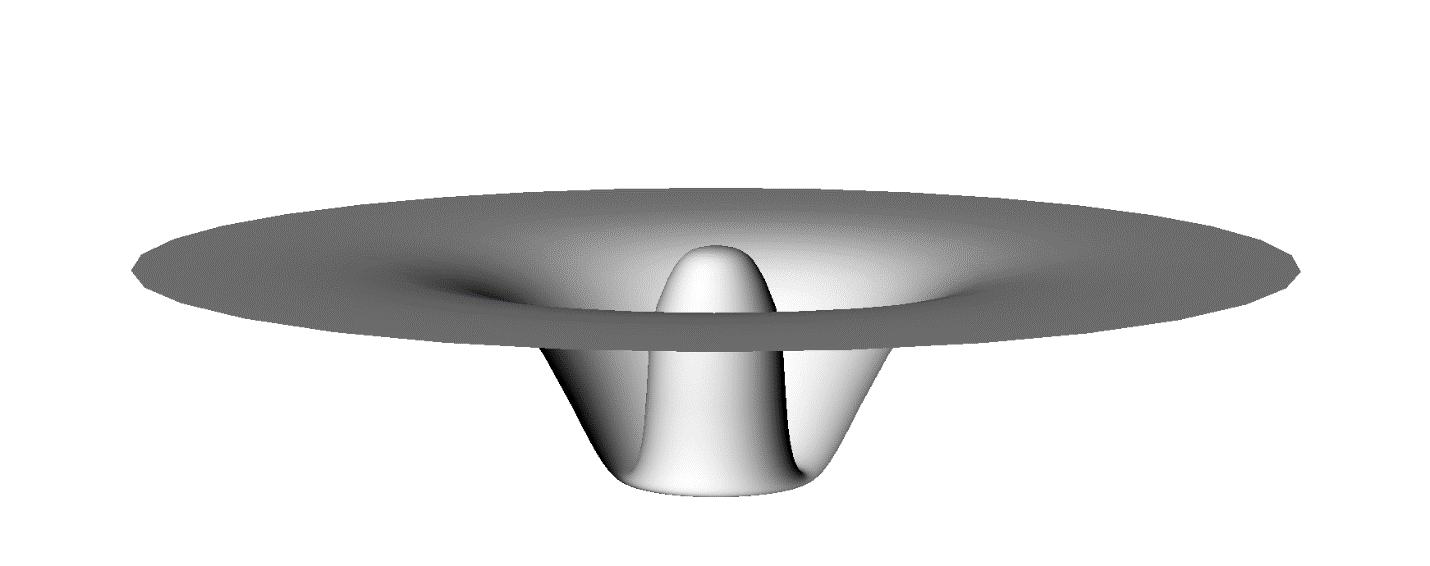Figure 1. Space-Time curvature due to a mass

In the curvature of space time as described by Einstein,objects are pulled into low points as illustrated by Figure 1. However, there is no mechanism described by Einstein to explain how this occurs.There is no space-time functional equation that results in a numerical force.It would seem that gravity pulls the objects into the well. Figure1 is a graphical representation of Newton's original work. This diagram of the curving of space-time implies that a ball would simply roll into the well. Placing a ball representing a simple mass would create an inconsistent model or relationship and would no longer be a physical cause and effect function. A ball would be a well.

Figure 2 illustrates the curvature of space-time that is represented by Newton's calculation which equates Force with a mass and distance quality. The curvature of space-time is an alternate descriptor of Newton's work, not a cause. There is nothing about the curve that would make a well move closer to another well. If there was something that pulled wells together, the effect of the wells merging would cause the universe to vanish into a single well. That would be entirely contrary to known observations.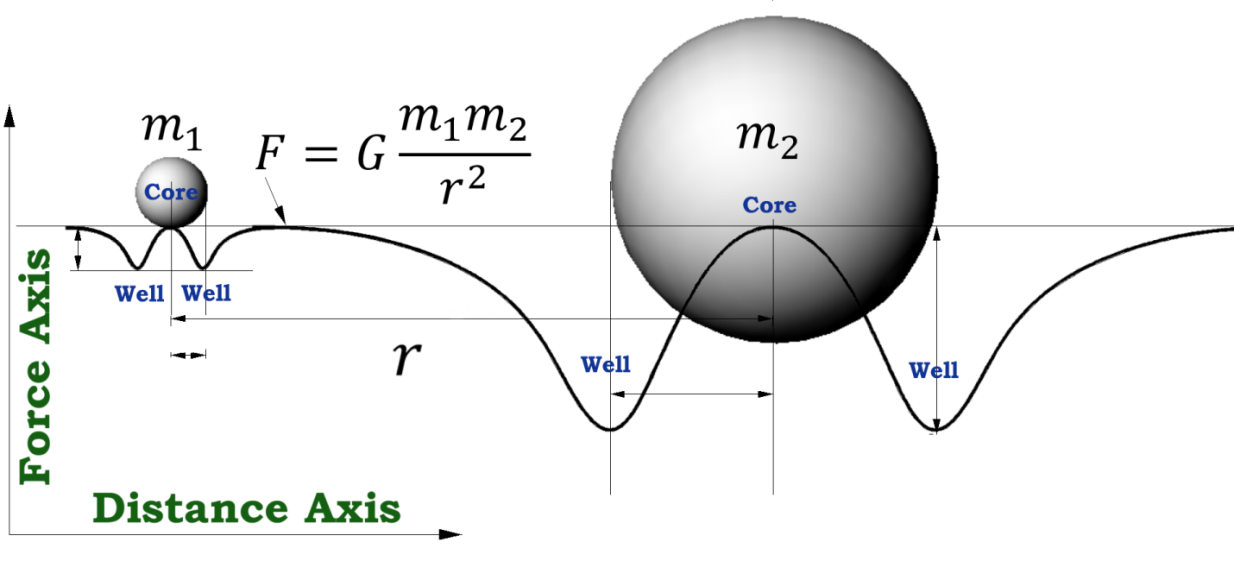Figure 2. Newton's forces

3. There is something invisible that pushes two masses together.Note that this invisible force could resemble the force created by a fan facing down over the space-time bowling ball model described above in Figure 1. However, that would imply aether exists, which is a troubling topic. The aether theory is based on the idea that there is a fluid that permeates space and supports the physical realm.

There are two problems with the theory presented as item 3: erosion of the material and inertia. If objects are pushed together, eventually the force of particle streams would erode matter into dust balls. To make this possible, these particles would have to come from all directions to permeate the matter in all directions, because gravity is directionally independent. If this occurred, these invisible particles and their interaction would eventually stop all matter and energy because there would be a net force as the result of the matter's motion in any direction. Eventually, this would bring all matter to a stop. This outcome is not possible because it violates an observable law of motion first stated by Newton: an object in motion will remain in motion until a force acts upon it.

4. There is an electromagnetic force that pulls or pushes masses together. Consider that motors, servos, high voltage, current, magnets, and a myriad of combinations of those elements have been configured and operational in the last 100 years. If gravity was related to electromagnetic phenomena, man would have stumbled on that fact during this long period of using these tools and devices.

The overall human effort involved in electricity and magnetism exceeds the man hours devoted both to exploring gravity described previously and exploring for the mass particle. For every hour the Large Hadron Collider operates, there are at least one million man hours devoted to electrodynamics. This equals more than a quintillion man hours of effort devoted to the topic.

Reasoning has discarded each of the four possibilities for gravity, as summarized below:

1. Springs connect everything: Nonsensical because it contradicts the inverse square law

2. The bending of space-time makes wells and mass falls into the wells: Infeasible because it simply redefines the problem of space-time bending and the question of gravity

3. There is something invisible that pushes two masses together: Problematic because it contradicts the first of Newton's laws. However, it does model gravitational effects

4. There is an electromagnetic force that pulls or pushes masses together: Impossible because interactions between gravity and electrodynamics have not been experienced by experts in the field.

Reasoning rules out the four possibilities, but, nevertheless, there must be a cause for gravity. Therefore, using reasoning, the next step is to reassess the problem.

# Neutrino Refraction

## Force interactions

Research can be used to look for both a cause and an effect of gravity. In this case, it may not be a strong effect, because gravity causes only a slight change when things are at rest. Compared to electromagnetics and other strong forces, it is not powerful. Gravity is not an interaction that changes matter or energy. What researchers are seeing and looking for is a different kind of interaction -- one of force, but no change.

There are four types of interactions:

1. Heisenberg matter collision effects

2. Einsteinium photoelectric effects

3. Energy colliding with energy causing the superposition of normal nodes

4. Matter colliding with energy resulting in disintegration or structural state changes like melting

All of the above interactions result in a change in the structure or state of the components. Gravity does not. Therefore these interactions should be ruled out.

There also is a subtle effect called refraction. This occurs when energy glances off matter and modifies its momentum vector. For example, light passing through water or glass. Neither is significantly changed, though there may be heating and a change in direction.

The idea of refraction is intriguing because neither party in the refraction is modified, only the velocity or kinetic energy changes. Blue light remains blue light when it passes through glass, though in passing through the glass, its velocity and direction may change.

Photons interact with matter and are energy. The simple quantum equation

E = hv

shows the relationship that is the basis of the photoelectric effect. It indicates that energy is proportional to the frequency of a photon. The famous equation E = mc2 also implies hv = mc2 and infers that light has a momentum property.

With refraction in mind, reexamine the four possibilities. In that case, numbers 3 and 4 show promise.Possibility 3, There is something invisible that pushes masses together, may work if there is an invisible particle that permeates matter and energy. Possibility 4, There is an electromagnetic force that pulls or pushes masses together, could be an option if there is a low-level universal electromagnetic property for all matter.

Optical refraction is an observable particle-matter interaction that will eventually result in changes to both particle and matter due to absorption. For an explanation of gravity, what is needed is a particle that refracts through matter but is not observable like photons are due to the photo-electric effect. Neutrinos meet this requirement.

## Neutrinos

Wolfgang Pauli's name is inseparable from his pioneering hypothesis of the existence of the neutrino. This was confirmed by experiment 25 years after he proposed its existence in the 1930's. The starting point for Pauli was the continuous energy spectrum of beta rays which could not be explained by a theory at that time. Niels Bohr attempted to construct a theory by stating a hypothesis that presented the restricted validity of the principle of energy conservation.

Pauli could not accept the Bohr Theory because the principle of the conservation of energy had been proven in all fields of physics previously. That proposition seemed plausible to him. He was anxious to provide a solution to this problem and proposed that during beta decay apart from the electron, a further, but electrically neutral particle, is emitted. During emission, the sum of the energies of both particles is constant. On December 4, 1930, Pauli wrote his famous letter to the "Dear Radioactive Ladies and Gentlemen" who gathered in Tübingen. In it, he sketched his idea and inquired how things stood with experimental proof of what he had experienced and postulated.

Further scientific endeavors and physics observations since that time have resulted in cataloging several types of neutrinos.

A muon decays into an electron with a 200:1 mass loss after a time delay of its creation of 2.1 micro seconds. No other particle can be detected.

It has been theorized that neutrinos travel through matter at considerable densities and at the speed of light. There is substantial evidence to implicate a possible refractive interaction between matter and neutrinos. In this case, what the neutrino lacks in mass, it more than makes up for in sheer density and has luminal inverse square properties like photons.

## Gravitational effects of neutrino refraction

If neutrinos are in fact as abundant as modern science portrays, the theory that they are coming from multiple directions is within the realm of possibility. If that assumption is true, it is reasonable to at least consider the possible effects of neutrino refraction with matter.

Consider a simple sphere of matter in space not near any other objects. Neutrinos will travel in all directions through it. Any resulting force of refraction would be a net of zero as shown in figure 3.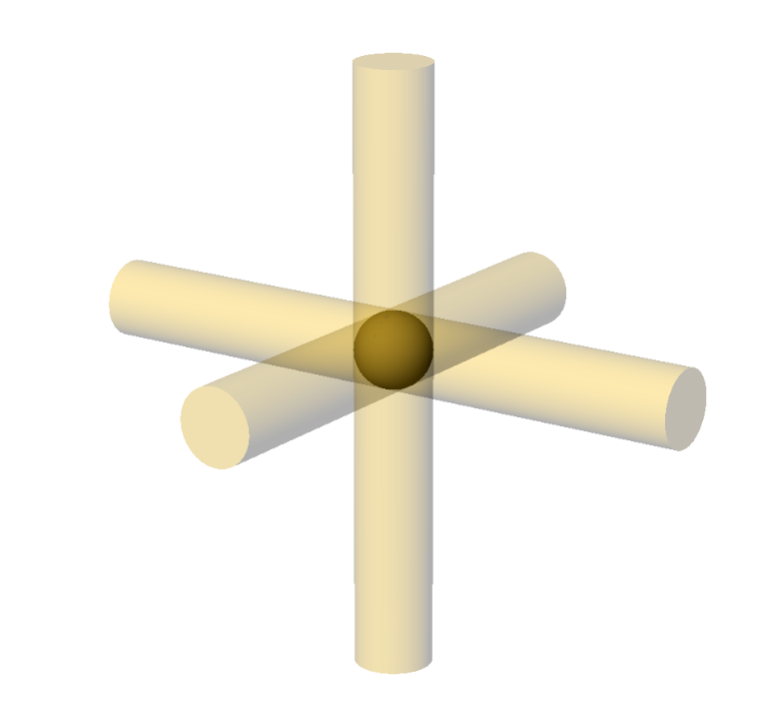Figure 3. The flow of neutrinos through a sphere of matter in freespace

In spite of that result, it is clear that the trajectories of the neutrinos would be disturbed. The matter would change their direction, just as a sphere of glass would change the trajectories of a beam of light. The effect would be to create a shadow of a sort, such as that displayed in Figure 4.

Consider two spheres at some distance from each other. Imagine a line between them, as displayed in Figure 4. This line is the direction that neutrinos will travel if only one object is present. Neutrinos will be present on either side of the line segment between them. For example, imagine a force of 1 experienced in two opposing directions towards the segment in between the two spheres. Because the neutrinos are disturbed after traversing through the spheres, the force they exert is less than 1when they encounter the next sphere in the line. In that case, there will be a net force exerted on the spheres in the direction facing them. The greatest imbalance of forces is where the shadow effects are the greatest and when the spheres are the closest. The further away the spheres are from one another, the less they will affect each other. The larger the spheres are, the more shadowing they produce. This mathematical relationship is expressed below Figure 4.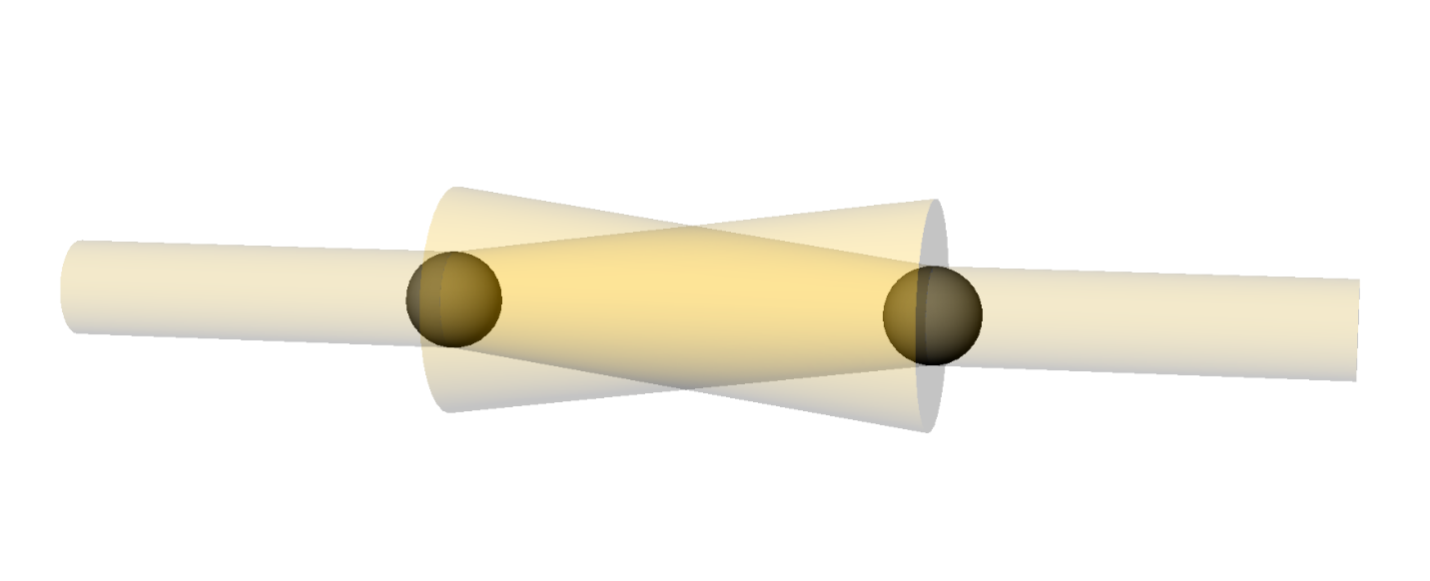Figure 4. The interrupted flow of neutrinos through two spheres shadowing each other

This equation is similar, or equivalent to Newton's equation expressing gravitational force. The larger the masses m1 and m2, the more the disruption of flow and the further away, the less they are affected by the disruption of flow in an inverse square relationship.

Another way to look at neutrino refraction with respect to gravity is by devising a simple hydraulic model. Imagine two garden hoses facing each other with the water flowing equally as either a stream or spray. Between them there is a mesh screen which resembles a window screen that hangs freely. If properly regulated, the two flows of water will keep the screen in position.

In this analogy, the flow of water represents neutrinos. The screen represents matter. The situation depicts free zero gravity space.

Now imagine another hydraulic model. Place another screen in the flow between one hose and the existing screen. In that case, the exterior flows go through the screens which would normally be at equilibrium. However, because the interiorflow has been disturbed, it does not flow as smoothly as the exterior flows. This situation results in the screens being pushed together until they touch. This effect demonstrates gravity. This analogy and model hold true for all geometries of gravity. If more mass is present, there is more disturbance.

It appears that of the original 4 possibilities for the cause of gravity, the third possibility, T here is something invisible that pushes masses together, is applicable and a realistic working model when considering neutrino refraction.

There is another consideration related to the neutrino refraction/interaction concept. If neutrinos are as energetic, prolific,and dense as is assumed by science, the interaction of neutrinos and matter would be violent. It is. When a single neutrino interacts with miles of ice like the ice at the IceCube South Pole Neutrino Observatory, one interaction produces photons that are visible to the naked eye because they are so bright. When this occurs, there are lumens and watts of intensity, not electron volts or micro-nano-ergs of force or minute amounts of energy. Instead, there is an amount of energy that is devastating to a microscopic realm.

Neutrino refraction does appear to be a viable explanation for gravitational effects. Relativistic effects also can be explained quite simply by the refraction of neutrinos.

## Relativity, the special case

The refractive model could explain what causes the effects of special relativity. It is possible that increased velocity of an object results in more neutrinos traveling towards that object increasing the refractive forces against it. As this occurs, there are fewer incidences or refractive effects away from the direction of travel because of the forward velocity. This may affect the acceleration of a material object, because the matter encounters an ever increasing density of neutrinos. It also would compress the material in the direction of travel.

Einstein predicted these effects of acceleration. They are the same effects that would be seen in the Doppler Effect, namely a shift in frequency of acoustic or electromagnetic radiation. The shift would be to higher frequencies when the source approaches and to lower frequencies when it recedes.

Relativistic time dilation is an interesting problem. It is not about time as a dimension but instead concerns delta time as a measure of change in the change in time. A clock's internal motion would slow as the apparent mass increased.

The Lorentz transformation mathematically defines accelerating dynamics, as stated in the special theory of relativity. The transformation states that the faster an object moves, the more force is exerted against it in the form of an ever increasing apparent mass. In other words, the mass or apparent mass of an object increases relative to the velocity. This is can be thought of as a space-time Doppler Effect.

If an object is encountering a frontal force due to neutrino refraction and less from behind, the faster the object moves, the greater the amount of increase in apparent mass. At the speed of light, an object encounters an infinite amount of force, or apparent mass, in the direction of travel.

This results in pushing against and effectively squeezing the object in the direction of travel, and weighs down any internal motion. This specifically affects clocks. Notably, time dilation, (slowing of time) has nothing to do with time travel. It refers only to the slowing of a local mass-based clock as it moves faster. The only clocks known at this point are based on the motion of a mass.

To grasp the nature of the neutrino strings that affect gravity and relativity, it is important to explain their dynamics and the effects of their interactions.

# Dynamics of Neutrino Strings

## Explanation of dynamics and inertia

There is one problem related to the belief that the refraction of neutrinos causes or results in gravitational and relativistic effects.That issue is inertia.

If the gravitational and relativistic effects are the result of a dynamic interaction that varies with velocity in any direction, eventually this would cause all matter to stop moving until the net forces exerted against the mass became zero. This violates the observed relationship that a mass in motion remains in motion until a force acts against it, one of Newton's Laws of Motion.

There are two possibilities.

1. Neutrino refraction is not a viable explanation of the gravitational and relativistic effects, or

2. There is an interaction between matter and neutrinos that allows refractive effects and supports inertia.

There is another similar rule or law that supports the notion of something in motion remaining in motion and has nothing to do with inertia. This is called inductance. When a coil of wire is formed and a current is passed through it, the magnetic field and the current flow is reluctant to change. This is due primarily to the magnetic field and not to the mass of the electrons or their inertia.

If an object moves, it causes a neutrino string to bind up or coil when the string is headed in the opposite direction of travel. This could explain observed phenomena. The faster the object goes, the more inductance occurs. This manifests itself in an increase in apparent mass, and keeps the object moving at the same speed.

The reactance of free space is 376 ohms. There must be a cause for this reactance. When neutrinos cross paths or nearly cross paths, they can bind up and coil. This coiling results in an inductor situation and may be the reason for the free space reactance. See Figure 5 below: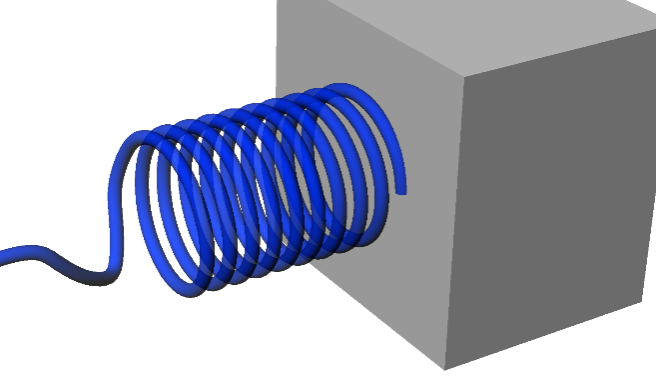Figure 5. Inertial induction

A neutrino string would explain a great deal. If neutrinos bond and form a chain or string, the string would tend to be inert to other things. It also would explain an h/2 relationship in quantum mechanics, as the coiling up of a linear string would result in a circular configuration.

These strings traveling at the speed of light also would be able to make a string pair of two opposite types of neutrinos that would form a twist or helix. There are many examples of self-confining plasmas and molecules in nature. It is reasonable to assume this occurs at the subatomic level, as well.

A self-confining twisted pair also would be at charge and at dynamic equilibrium. In other words, they would be stable and balanced systems.

With an effective model of gravitational and inertial effects, neutrino refraction appears to be a valid concept to explain gravitational effects. It is effective and valid because it implies a direct relationship between neutrino interaction, mass, and a resultant force. The net flow of neutrinos per second required to create one Newton of force, F, on a one kilogram mass, M, is one Berne, B.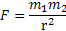## Latent inertial induction

The general theory of relativity implies a concept of inertial frame drag. This wake was experimentally verified by NASA's Gravity Experiment B. The effect can be modeled simply by neutrino string induction.

When the neutrino strings that are coiled up on the trailing edge of an object in orbit are left with no obstruction due to the objects trajectory and motion, the coil unwinds and eventually becomes a straight string. During this process, there is an inertial induction force with no apparent cause, or latent induction, which is perceived as a gravitational wake.

This same mechanism of inertial frame drag can explain the situation depicted in an article in the February 2014 edition of Scientific American titled The Proton Problem. Simply stated, a different diameter of the proton exists under two different circumstances. The diameter of the proton is smaller when it is the center of Muonium, orbited by a muon, than when it is orbited by an electron in hydrogen.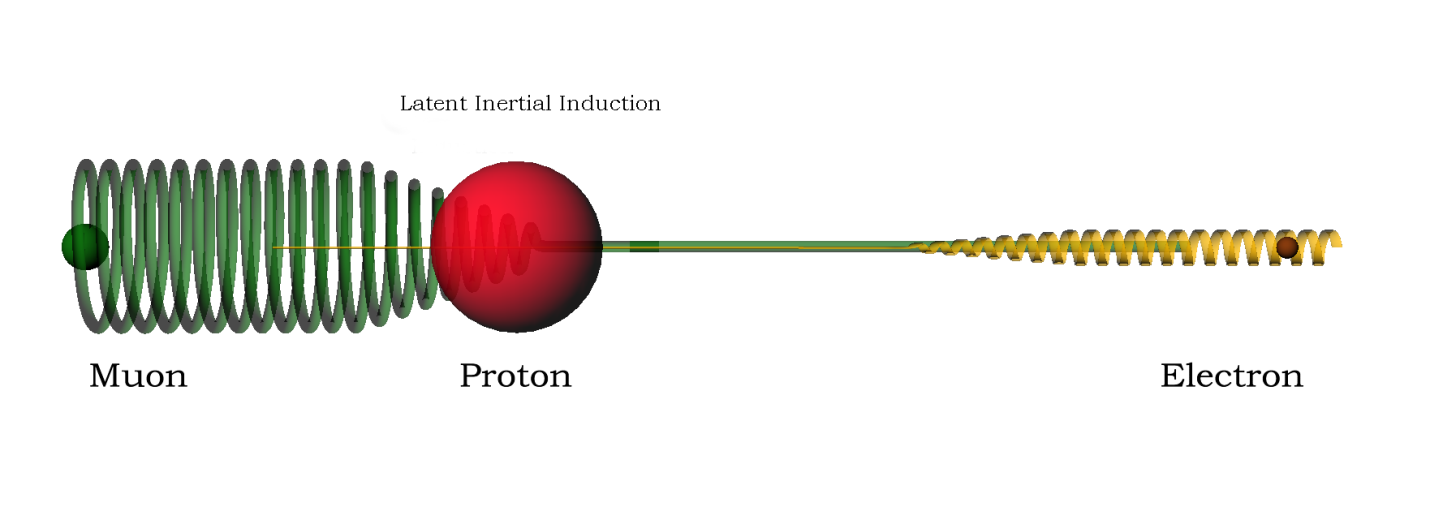Figure 6. Comparing the latent inertial induction of an orbital muon and an electron

In hydrogen, the electron's orbit creates the same inertial frame drag as in a planetary example, but on a much smaller scale. Before the neutrino string coil can reach the proton, the coil unwinds intercepting the proton with no inertial induction and passes freely through the proton.

When the neutrino string coils are freed to straighten out, they require a certain amount of time and space to do so. That amount of space is greater than the distance between the muon and the proton in Muonium. As a result, an inertial push is exhibited on the proton, causing it to be compressed and therefore smaller.

## Representation of neutrino string entanglement

An interesting interaction demonstrating entanglement can be demonstrated with two long rubber bands. Twist each one, one in one direction and one in the other direction.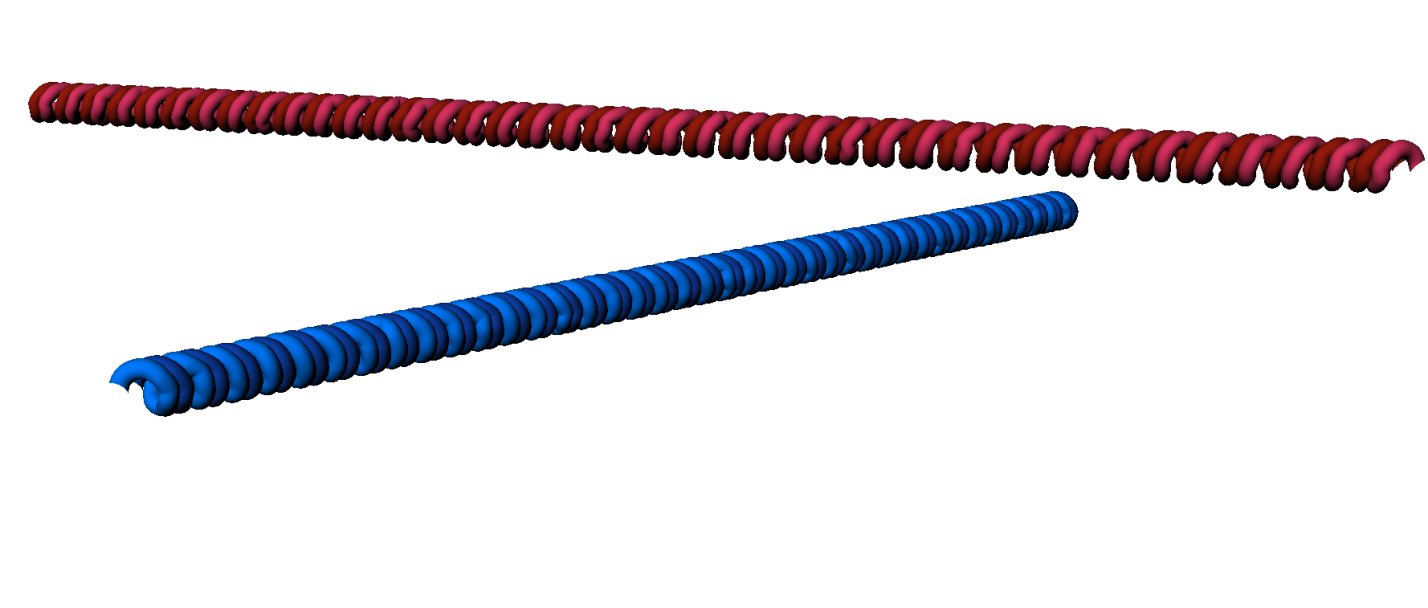Figure 7. Two neutrino string systems with opposite twists

Both bands are tightly wound and start forming double twists. Stretch both bands and hold them against each other lightly at a 90 degree angle.Figure 8. Perpendicular overlap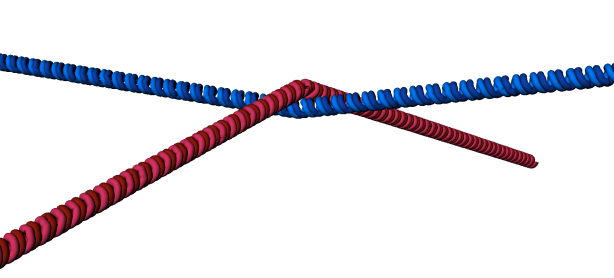Figure 9. Intersection and overlap

An imaginary line between the end points should cross or pass through each other as they are pressed against each other's middle point area. Next, relax the bands. When relaxed, the strings intertwine, or entangle, at an angle normal to and between each other.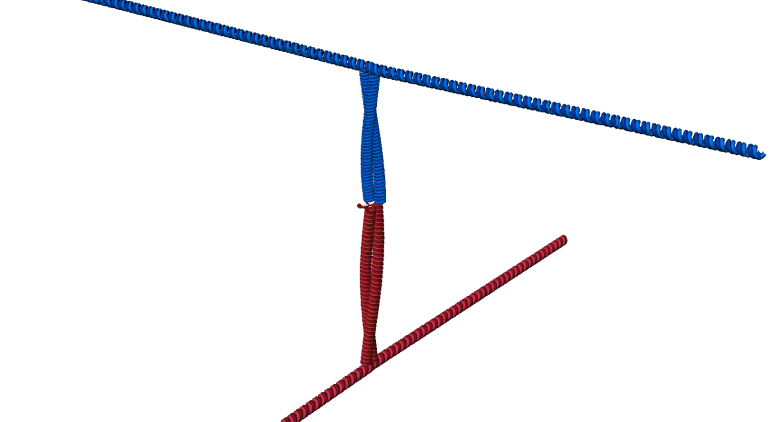Figure 10. Neutrino string entanglement

Relaxing the bands has three outcomes:

1. Mutual cohesion between different string rotations

2. Creation of a third string normal to both

3. Interaction of two strings occurring as they change tension and demonstrate a transfer of forces from one string to another

One may characterize this interaction as entanglement in its simplest form.

The two different string systems impede each other. It is notable that when the two bands are twisted in the same direction, they do not intertwine. In fact, in that case, the two bands appear totally inert to each other and do not impede.

The entanglement described above causes cohesion between different systems or configurations of neutrino strings. There is a common cohesion called Charge.

## Charge

Electrical Charge has been observed since the dawn of mathematics. Charge comes in two forms: plus and minus. Most likely, charge is responsible for one of the first tools in man's history when the electrostatic discharge of clouds ushered in the age of fire.

Understanding the relationship between charge and a magnetic field came much later in 1820 when Orsted noted that when a charged particle moved through space, it generated a magnetic field. When a magnetic field changed, the charged particles moved.

Similarities between the features of a magnetic field and the superposition of normal modes of a two point emitter are striking. The lines of force and the connect-the-dots lines of nodes and nulls in a two point emitter's field are similar. It appears that there is a relationship between them, or that they operate through a similar mechanism.

If neutrinos make strings by linking ends together due to electrical charge, neutrinos in the form of strings or self-confining helical structures might themselves exhibit charge if they are not exactly balanced. For example, if a loop of neutrinos has 25 plus and 24 minus neutrinos, a net of plus one would result. Or strings could form spheres or spherical shells. If so, there is the possibility of a structure that is electrically balanced that exhibits an exterior charge. This occurs because the counter charge's surface contained in the interior of such a sphere would be observed to be zero due to the inverse square law. This structure also would explain quantum spin taking place as a result of the neutrino's motion within each layer. Charged neutrinos may play a role in forming matter due to their dynamics and motion.

## Particles and nuclear structures

The theory that neutrino strings form matter seems to be a difficult proposition at first. Such a structure logically would be spherical due to even pressure or stress. Particles may be layers or shells of these neutrino structures. (Refer to Figure 11) If successive layers are attached or confined due to a charged surface structure, when these shells are disturbed or decay, the resulting by-products would show a conservation of charge and spin. This also would explain why in virtually all such decay modes of particles a neutrino seems to a by product.Figure 11. A layered structure of charged shells

Consider the neutron. When left to itself, it decays into an electron, a proton, and an anti-neutrino. Why? Perhaps a neutron is a proton that is covered with a shell that is comprised of the neutrino configuration that forms an electron. The anti-neutrino may be the fastener that keeps the neutron together, or the odd piece of the outer shell that is left over. This would explain the odd nature of the atomic nucleus. If the surface skin is shared between a neutron and a proton, as shown in Figure7, for Deuterium, it would form a stable structure.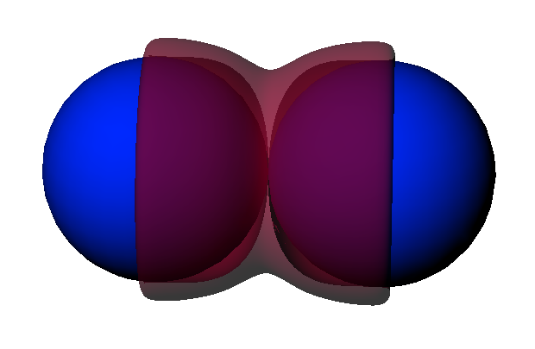Figure 12. The element Deuterium

Deuterium's structure displayed in Figure12 is balanced and stable. This also could explain why the gluon particle theory is so widely accepted in nuclear chemistry, although no one has ever found a gluon. It simply is a state of the electron's existence and a state that only can exist in the nucleus. It is like a taut rubber band that is stretched around something which, when not in use, is simply a rubber band.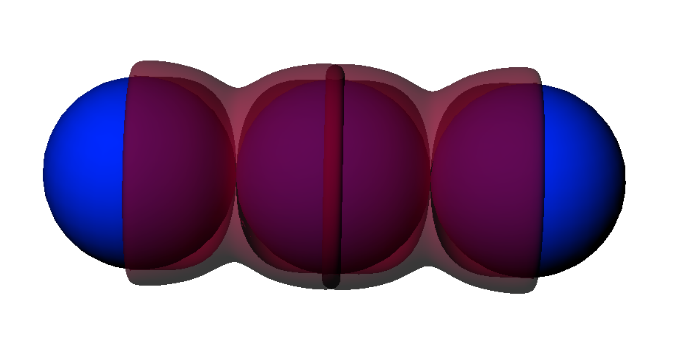Figure 13. Tritium with the electron/gluon overlapFigure 14. Tritium and Tritium overlap region outlined

Figure13 is a diagram of the element Tritium. An overlap area has been outlined in the Figure 4 to expose what could explain Tritium's radioactive instability. It does not seat or fit perfectly like the deuterium nucleus in Figure 12. Because of this looseness or slight internal pressure, the nucleus eventually falls apart and eliminates the extra ill-fitting neutron.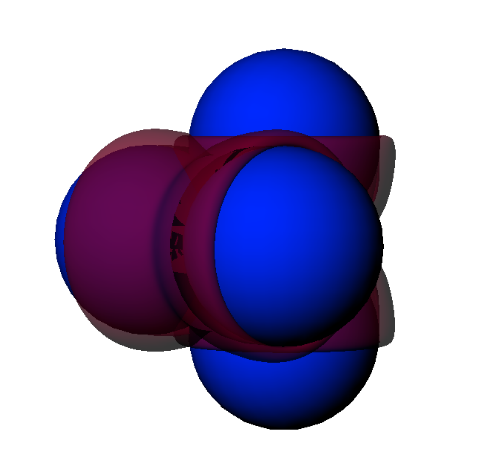Figure 15. The element Helium

Figure15 is a model that shows Helium as a tight fitting and balanced nuclear system. In Figure 15, the electron/gluon fits evenly and holds the protons snugly by creating a stable configuration similar to the Deuterium example above. This explanation is the missing piece of the Bohr Nuclear Model. Examining the neutrino's refractive effect on elements has broad implications for nuclear particle decay and nuclear stability.

## Nuclear decay and electron transitions

There is no effective and conclusive reason for two common and observable phenomena: electron orbit changes and nuclear decay. We do understand what these phenomena are and can describe them with amazing accuracy. However, how and why have yet to be discovered.

Neutrino refraction could explain a great deal about nuclear and particle decay, and stability. It could be the invisible nudge that pushes the unstable configuration over the edge and yields the stable by-product structures, as well as the cause of electron orbit transitions.

An electron goes from an energetic state to a lower energy state in atomic orbits and radiates a photon. The energy change is represented in the frequency of the photon. However, it is unknown what causes the electron to go to a lower state.

Scientists also understand that nuclei undergo a statistical decay called a half-life. Yet they do not know the cause of the decay. It is notable that neutrinos are involved in almost every decay mode of every element. This has been viewed as a fluke curiosity, but instead should be considered a dynamic component of the process.

There is an inconsistency among half-life decays, as illustrated below in the Periodic Chart of the Elements, Figure 16. The metals of extremely long half-life decays are circled in blue. However, one, Technetium 43, which is virtually dead center among all those metals and circled in red, is an element that has one naturally occurring stable isotope, all of which decayed long before the advent of man. The other Technetium isotopes are unstable and short lived on the order of seconds to months.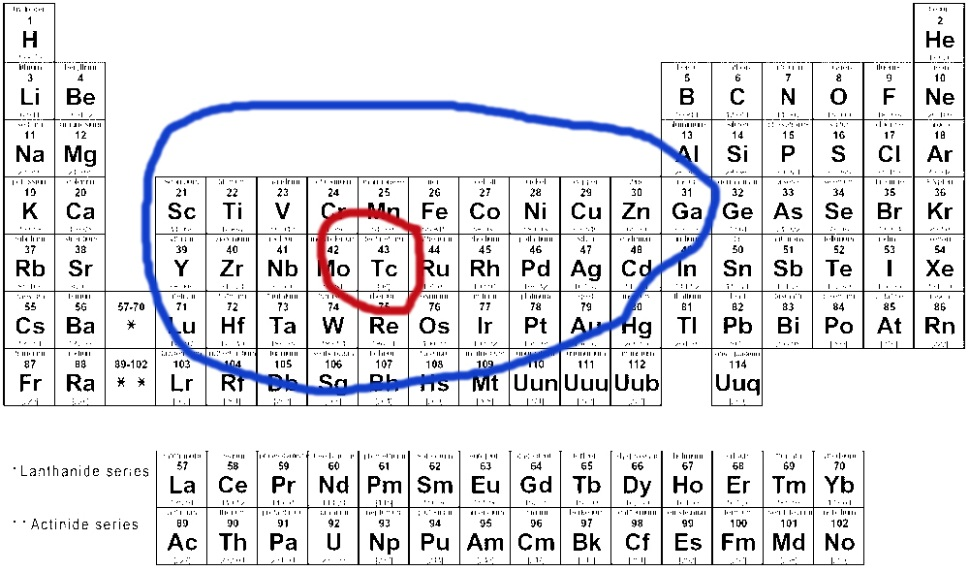Figure 16. Periodic Table of Elements and Technetium's neighboring elements

It could well be that Technetium's structure makes it particularly vulnerable to neutrino refraction and this makes it easily disturbed into a nuclear decay mode. Neutrinos may well have effects at both the minute nuclear level and at a larger, more observable level.

# Macro Effects of Neutrino Refraction

Gravity is in fact very simple --it makes things fall and sometimes break. It is a huge burden and the daily cost of fighting it and its cascading effects are the drudgery of man's existence. It has little to offer other than keeping things from floating away.

There are a few things that are interesting because they seem odd, inconclusive, or mythical and may have a basis in fact and be related to neutrino refraction. These macro effects could include the following phenomena.

## Taos Hum

Some witnesses report hearing a hum that is un-localizable. It is recordable but no source can be found. There are places all over the world that have reports of this phenomenon. Could this be a result of neutrino refraction with interference or a beat frequency?

If two streams interfered with each other, there could be an underlying oscillation. Even if the interference with neutrinos is slight, this could result in a large scale observable vibration. Even a slight variation of the strings or flow could result in a measurable vibration.

## Neutrino string induction and the Big Bang

The idea that the universe started with a big bang is fundamentally sound. Dr. Stephen Hawking first proposed the idea. After all, it appears on the face of things that there was a starting point and that the universe arose from that event.

So what was the event or cause?

Start with the idea that neutrino strings are, as described above, two neutrino flows that form a self-containing/confining helix plasma.

Imagine that there were two neutrino balls of string that collided and intertwined; as depicted in Figures 7-10. This is what Dr. Stephen Hawking referred to as the Singularity. One gigantic string may haverapidly expanded into a huge circle and subsequently stopped, once it finally unwound. If there was a complementary string that expanded, the two strings could have interfered with each other's expansion and slowed down expansion. This model resembles the two oppositely wound rubber band example explained in the section above titled Representation of neutrino string entanglement.

If this occurred, the strings could have inhibited a rapid expansion by twisting against each other, expanding outward and unraveling at a considerably slower rate than a single string.

Some interesting things might have occurred during the Big Bang if this theory is true. At first, in massive chaos these strings could have clung to each other and formed twists. They might have expanded at the speed of light in all directions like a spring by crossing, looping, and eventually forming the particles we call matter. Eventually, after this chaotic start, the mass could have become more stable and kept expanding but more slowly. Later, it could have evened out and for a long time expanded as amass of stable secondary structures or shells forming what is called matter.

## Gravity Lensing

If the universe is in fact comprised of a three dimensional neutrino string web or fabric, it is not unreasonable to assume that the gravity effects around a galaxy would cause light particle trajectories to be slightly altered. Light particles from one galaxy could be pushed around a closer galaxy and appear to make a ring. Einstein predicted this. The effect has been observed and recorded on many occasions.

## Neutrinos and dark matter

If a three dimensional structure can be manifested in space, it is possible that a simple loop of neutrinos can exist. These loops of simple neutrino strings could be partially detached from the rest of the universal neutrino flow. If large areas of these closed loop structures exist, they might interfere with the galactic flow. This interference could cause the appearance of a gravity force which would support the supposition of the presence of matter. Actual matter would not need to exist.

This might also explain the 4 degree Kelvin background radiation, as suggested by a British colleague Richard Day. While some think this is a remnant of the Big Bang, It should be noted that this radiation persists as if it is coming from an unidentifiable source, much like the Taos Hum.

## Neutrino manifolds and wormholes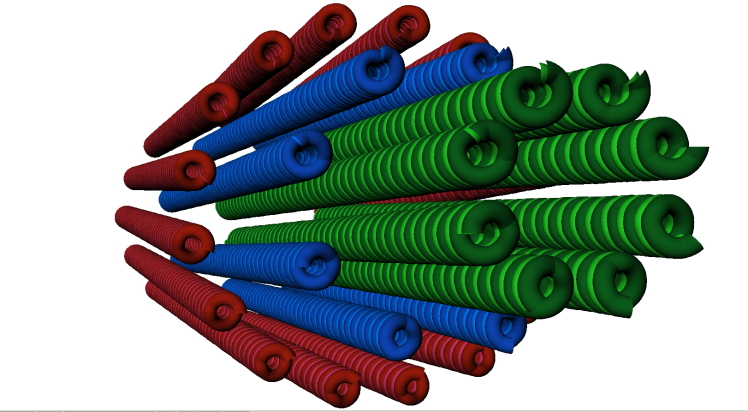Figure 17. A neutrino manifold

If a group of strings were to form a bundle with a core string, as shown in figure 17, would the inner string travel at the same speed as the outer group of strings? Or would the inner string travel twice as fast? Would the inner string piggy back on the flow of the outer core that it translates against or through? If so,it is important to ask if bundles of neutrinos allow for binary doubling of the speed of neutrinos (vn) to v n2x, and continue to multiply. Could this happen naturally or be created and form what is commonly referred to as a wormhole or manifold?

## Entropy

If a one subscribes to the idea that two strings are inhibiting the expansion of each other, as time passes, the inevitable will happen. They will twist and twine into a gigantic ring without kinks, twists, loops, or knots. The outcome will be a single gigantic ring with no material shells or structures. This may already be observable as what is called dark matter as discussed above under the section Neutrinos and dark matter.

If neutrinos form strings and shells, they also may form string loops or circles that would be isolated from the normal universal flow yet impede the flow. However, the string loops might not interfere with normal matter. Evidence of their existence would be a gravitational phenomenon without the presence of normal particulate matter.

These loops may be the occupants of the voids between large galactic clusters. They also may be beneficial to the neutrino flows that enter into galactic clusters. Encountering these loops of inert neutrinos may provide entropy, calming or smoothing regions of the voids and allowing the flow to refine and lessen any disturbances.

## Black holes and neutrino refraction

Contrary to popular opinion, black holes are not high gravity all-consuming giants. Along their axis of spin there are particle jets. The existence of particle jets is contrary to a theory of the unidirectional pull of gravity and also is an indicator that gravity is caused by neutrino refraction. If there was a unidirectional mass attraction as thought of in classical mechanics,these particle jets simply could not exist.

The neutrino flow through matter, particularly a star, becomes more and more disturbed as it begins to permeate matter. At some point, the disturbance is so profound that the flow is reduced to almost nothing compared to the incoming free space neutrinos that arrive in a straight line and are unfettered by any disturbance.

If the material in the star is rotating around an axis, the additional effect of latent inertial induction would increase geometrically the gravitational effects and an avalanche of matter would head into the core of the star. This can, in addition to compressing matter in the direction of travel, also slow down, or impede motion, causing a slowing of the internal clocks, as predicted by Einstein.

When the additional amount of inertial inductance becomes high enough, it creates a geometric collapse in the ability for exiting trajectory neutrinos to leave the object mass. This makes it impossible for material to leave the object's surface at the equator. This, however, would not happen along the axis of spin, creating a region for high energy particles to exit. These are the particle flares at the poles.

This situation could support the creation of large loops of neutrino flow that rotate around an axis. The more this looping happens, the more likely it is that the incoming flow will meet lesser and lesser opposition. This could create a situation where nothing exits at the equatorial region of a star because the incoming neutrino flow is unmatched. All matter around it would be pushed into the star.Figure 18. A black hole with particle jets at the poles

Figures 18 illustrates that particles that normally exit the star as energy particles meet the normal forces of free space and form particle jets at the poles.

# Applications of Neutrino Refraction and Neutrino String Manipulation

Neutrino refraction and neutrino string manipulation can be applied to engineering designs and solutions. This new field of Neutronics might pave the way to the following eight applications, as well as others not yet identified:

## Artificial gravity

Gravity is a result of a differential neutrino flow. Refraction is a change in the net vector of flow or index of refraction of neutrinos in an object. This results in a change in the net forces on an object. In free space, refraction results in either an acceleration or a net force exerted upon an object.

If the apparatus that made this modification in free space was part of the object, it would move the object. If there are two systems in free space, an artificial gravity field or zone could exist under certain conditions. For example, if a secondary apparatus was applied perpendicular to the original apparatus, and the apparatus was focused on objects within the target area's object, (with the original apparatus compensating for the lateral push of the secondary apparatus), the outcome would be an artificial gravity field.

## Anti-gravity

If the same apparatus as defined above were to be employed in a direction against the free space flow on a planetary sized system, the free space differential forces would be lessened. If enough effort by the apparatus was applied, the free space differential might be nullified, resulting in a zero gravity free space equilibrium condition. More effort would result in a net force for leaving the planetary object.

## Force fields

An apparatus that focuses or changes the neutrino flow in a given direction and has a large area would push against any object in its path. An opposite flow from the apparatus would compensate for the recoil force and stabilize the apparatus. An inertial holographic inductor could create forces with seemingly no source.

## Neutrino Piezo-Electric Transducer or the Zero Point Module

If neutrino refraction is responsible for the Taos Hum, the possibility of making a piezo-electric power generator that is stimulated by neutrinos is a viable possibility. This power source would be inexhaustible. Its limit would be based on the scope of the arrays or the size of the transducer.

## Super luminal speed communication

If a manifold of bundles of neutrinos can be sustained, it might be possible to encase manifolds in layers. Each layer could double the translation of the adjacent layer. If so, 16 layers would result in communication speeds of 65,000 times the speed of light. That means the time delay from earth to Pluto would be one half second. Thirty-two layers would be a 4 billion times faster. That would be essentially 144 light years in one second.

## Transporter

By creating a well-defined and regulated flow of neutrinos in one direction, it may be possible to create a near infinite force that moves matter at the speed of light to another synchronized regulation station or platform. A closely defined beam could actually be configured to perform fine and accurate work such as molecular manipulation and removal. Two such beams with opposite flows could simply swap matter from two locations.

## Communication

A neutrino pipe could contain another neutrino pipe; others could be added. Each successive boundary could result in a binary increase in the speed of light and enable communication at velocities above light's velocity. For example, by modulating the flow of the inner pipe, information could be sent to a receiving apparatus at binary multiples of the speed of light.

## Star Gate

A neutrino pipe containing another neutrino pipe and repeating that continuum could enable each successive boundary to achieve a binary increase in the speed of light. It would be possible to go great distances at speeds above light on the order of2to10…20..30 and additional geometric progressions.

# Conclusion

We know little about neutrinos because of their nature. The fabric of space time may in fact be woven in a three dimensional fabric of neutrino strings which travel at the speed of light in all directions. Pauli, Dirac, Planck, and Einstein believed that there was something unknown and unaccountable in astrophysics. They conducted research and inferred the presence of unknown particles and circumstances. This paper proposes that the unknown and perplexing particles are neutrinos in strings and that neutrino refraction is the mechanism that explains much that was previously mysterious.

Theorists generally believe that the universe as we know it started in a small ball of neutrinos and unraveled. This paper offers a theory about the mechanism of reality based on that belief modified to include a conjecture that the universe's beginning was with two neutrino balls, one with a plus charge and the other with a minus charge. This paper provides an explanation of physical phenomena that are called the laws of physics with reference to the neutrino refraction of those charged elements. The underlying model is based on reasoning that refers to and explains observed physical phenomena and theorizes about the causes for and behavior of these minute particles.

This simple concept models the following physical phenomena:

• Gravitational forces
• The change in apparent mass and length as an object is accelerated as predicted in the Lorentz transform equations and the special theory of relativity
• The first law of motion
• Inductive reactance of free space
• Inertial frame disturbances as predicted in the general theory of relativity
• The proton problem
• Electron orbit transitional causation events
• Nuclear decay causation and structure
• Gravitational lensing
• The nature of black holes.
• The nature of dark matter

The neutrino refractive string model is suitable as an explanation of these phenomena based on sound reasoning and the knowledge of physics to date. It is a reasonable next step for the scientific community to examine the ideas presented in this document on their merits and to conduct research to further explore this topic.

# References

Berne, R. (2014). The Gravitational Effect of Neutrino Refraction. Library of Congress

Berne, R. (2014). The Relativistic, Gravitational and Inertial Effects of Neutrino Refraction, A Qualitative Model. Library of Congress

Bohr, N. (1921). Atomic Structure.

Einstein, A. (1916). "Relativity: The Special and General Theory" (PDF). Retrieved 2012-01-23.

Einstein, A. (1905), "Zur Elektrodynamik bewegter Körper',Annalen der PhysikSee also: English translation.

Einstein, A. Relativity: The Special and General Theory (1920), Chapter 11, The Lorentz Transformation.

Hawking, S.W. and Penrose, R. (1970). The Singularities of Gravitational Collapse and Cosmology, Published 27 January 1970. DOI: 10.1098/rspa.1970.0021.

Newton, Sir Isaac.Principia.Newton's Axioms or Laws of Motionpg. 19 of Volume 1 of the 1729 translation of Principia.

Orsted, H.C. (1854), Der Geist in der Natur.

Pauli, W. and Jung, C.G. (1955). The Interpretation of Nature and the Psyche.Ishi Press.ISBN 4-87187-713-2.